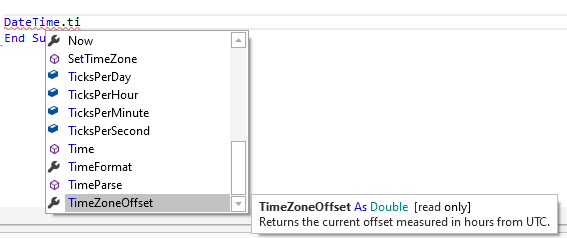# Android QuestionDateTime.Now problem with time zones

#### Arf

##### Well-Known Member
Longtime User
Does DateTime.Now return the ticks since 1/1/1970 to GMT time or UTC, or to the time on the device in question?

I send the current time to my external device for storage, like this:

B4X:
``````'now convert the time into it's parts
Dim timenow As Long = DateTime.Now
Dim calTime As spiroTime
calTime = Types.getDateFromTicks(timenow)
buf = Array As Byte(calTime.s,calTime.m,calTime.h,calTime.d,calTime.mo,calTime.y)
out.WriteBytes(buf,0,6)``````

The function called looks like this:

B4X:
``````Sub getDateFromTicks(intime As Long) As spiroTime
Dim outtime As spiroTime
outtime.y = DateTime.GetYear(intime)-2000     'DateTime works from 1970, my device dates work from 2000
outtime.mo = DateTime.GetMonth(intime)
outtime.d = DateTime.GetDayOfMonth(intime)
outtime.h = DateTime.GetHour(intime)
outtime.m = DateTime.GetMinute(intime)
outtime.s = DateTime.GetSecond(intime)
Return outtime
End Sub``````

Then at some stage I read the stored date back from the device:
B4X:
``UnitInfo.Calibdate = Types.getDateInTicks(pk.pData(52),pk.pData(53),pk.pData(54),pk.pData(55),pk.pData(56),pk.pData(57))``

The function looks like this:
B4X:
``````Sub getDateInTicks(s As Int, m As Int, h As Int, d As Int, mo As Int, y As Int) As Long
Dim result As Long
result = s * DateTime.TicksPerSecond
result = result + ( m * DateTime.TicksPerMinute)
result = result + (h-1) * DateTime.TicksPerHour
result = result + (d-1) * DateTime.TicksPerDay
Dim mn, yr As Int
mn = mo-1
yr = y+30        'DateTime works from 1970, my device dates work from 2000
Return result
End Sub``````

It all works fine for me in the UK, but someone is testing my app in the USA. Their tablet's time is set 12PM, EST in settings.
They store the time at 12:02, and it reads back as 06:02.

I'm having a bit of a struggle wrapping my head around it.

#### Arf

##### Well-Known Member
Longtime User
Ok so now I understand DateTime.Now is always UTC time.
So, I would like to keep storing the date in UTC, and convert the ticks that I read back, to local time.
I changed my function but it doesn't work:
B4X:
``````Sub getDateInTicks(s As Int, m As Int, h As Int, d As Int, mo As Int, y As Int) As Long
Dim result As Long
result = s * DateTime.TicksPerSecond
result = result + ( m * DateTime.TicksPerMinute)
result = result + (h-1) * DateTime.TicksPerHour
result = result + (d-1) * DateTime.TicksPerDay
Dim mn, yr As Int
mn = mo-1
yr = y+30        'DateTime works from 1970, SpiroConnect works from 2000

'now we need to adjust between UTC and local time
Dim diff As Long

'this should convert UTC time to a string rep of local time and then back into ticks, right?

diff = DateTime.Now - DateTime.DateParse(DateTime.Date(DateTime.Now))
result = result + diff
'done

Return result
End Sub``````

So the question is, how can I get the tick value for local time?

#### Andrew (Digitwell)

##### Well-Known Member

Also try using the timezoneoffset method•Arf

#### Arf

##### Well-Known Member
Longtime User
OK so I changed that function to this:
B4X:
``````Sub getDateInTicks(s As Int, m As Int, h As Int, d As Int, mo As Int, y As Int) As Long
Dim result As Long
result = s * DateTime.TicksPerSecond
result = result + ( m * DateTime.TicksPerMinute)
result = result + (h-1) * DateTime.TicksPerHour
result = result + (d-1) * DateTime.TicksPerDay
Dim mn, yr As Int
mn = mo-1
yr = y+30        'DateTime works from 1970, SpiroConnect works from 2000

'now we need to adjust between UTC and local time
Dim diff As Long
diff = DateTime.TimeZoneOffset * DateTime.TicksPerHour
result = result - diff
'done

Return result
End Sub``````

Now I am much closer - one hour out! Which must be something to do with daylight savings then. I can't see any settings for daylight savings on my tablet though.

#### Erel

##### B4X founder
Staff member
Longtime User
•makis_best and Peter Simpson

#### Arf

##### Well-Known Member
Longtime User
Thanks Erel.
This is working now...
B4X:
``````Sub getDateInTicks(s As Int, m As Int, h As Int, d As Int, mo As Int, y As Int) As Long
Dim result As Long

'convert the time to a string
Dim timeString As StringBuilder
timeString.Initialize
timeString.Append(NumberFormat(d,2,0)).Append(":").Append(NumberFormat(mo,2,0)).Append(":").Append(NumberFormat2(y+2000,4,0,0,False)).Append(" ")
timeString.Append(NumberFormat(h,2,0)).Append(":").Append(NumberFormat(m,2,0)).Append(":").Append(NumberFormat(s,2,0))

Dim store As String = DateTime.DateFormat
DateTime.DateFormat = "dd:MM:yyyy HH:mm:ss"
result = DateTime.DateParse(timeString.ToString)
DateTime.DateFormat = store

Return result
End Sub``````

#### Erel

##### B4X founder
Staff member
Longtime User
Why aren't you using DateUtils.SetDateAndTime???

#### Arf

##### Well-Known Member
Longtime User
I somehow missed seeing that functionI know for next time now! Thanks.

Replies
5
Views
1K
Replies
7
Views
664
Replies
7
Views
1K
Replies
11
Views
4K
Replies
106
Views
92K